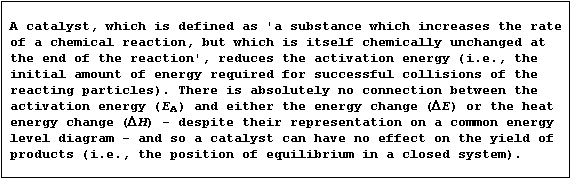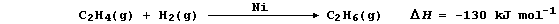```SELECTED PRINCIPLES: CHEMICAL ENERGETICS (2)
Regardless of whether a chemical reaction is exothermic or endothermic,
energy is required to break bonds of the reactants before those of the
products are formed. So, for a reaction to occur, the particles must
collide with sufficient energy for these bonds to be broken; this
minimum energy is known as the activation energy.
Shown below are two pairs of energy level diagrams: the first shows the
the general characteristics and an example of an exothermic reaction;
and the second shows the general characteristics and an example of an
endothermic reaction.``````1.  Hydrogenation is often used to convert unsaturated compounds, which
contain carbon double or triple bonds, into saturated compounds, which
contain carbon single bonds. The symbol equation for the Ni-catalyzed
hydrogenation of ethene to (saturated) ethane is:``````Complete and label this energy level diagram for the above reaction.

Energy
___

___

_________________ _ _ _ _ _ _ _ _ _ _ _ _ _
_________________

_________
_________

___________________________________________
Path of reaction

An important commercial application of hydrogenation is that used in
the manufacture of margarine, where unsaturated vegetable oils are
converted into saturated fats. The rate of this conversion is increased
by the use of a Ni-catalyst, which reduces the activation energy, and a
fairly high temperature (170°C), which increases the kinetic energy of
the particles so that more have the required activation energy for
successful collisions. Suggest one disadvantage of using an even higher
reaction temperature. _________________________________________________

Name two vegetable oils used in the manufacture of margarine. _________
_______________________________________________________________________

2.  Hydrogenation is but one of many examples of chemical reduction;
another is that of dinitrogen to ammonia:````This equation can be written as:````Show that the heat energy change (DH) for the above exothermic reaction
is -92 kJ mol-ą, by completing the Table using these bond energies:
946 kJ mol-ą (NşN); 432 kJ mol-ą (H-H); and 389 kJ mol-ą (N-H).```
 ` Bonds broken ` ``` Energy absorbed / kJ mol-ą``` ` Bonds formed ` ``` Energy released / kJ mol-ą``` ` ` ` ` ` ` ` ` ` ` ` ` ` ` ` ` ` Total = ` ` Total = `
```                                                                    
State the amount of heat energy released to the surroundings when
1 mole of ammonia is formed. __________________________________________

Dr. R. Peters                   Next                     Contents' List```